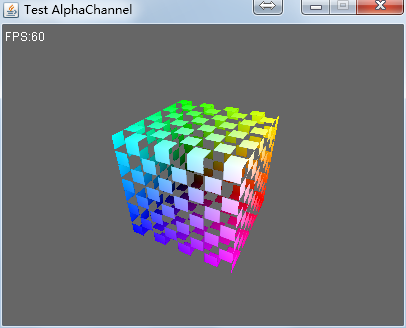## 目标

• 实现纹理透视校正插值（Perspective-Correct Interpolation）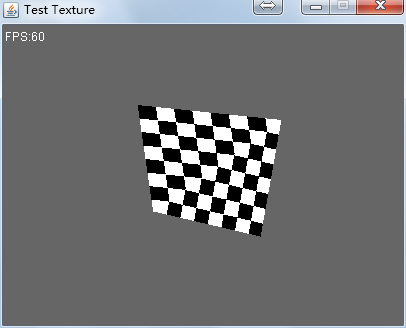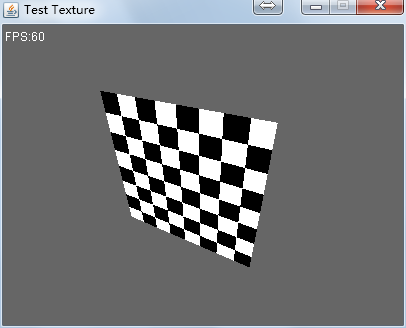## 实现

### 原理

``````x = (1-t)*x0+t*x1
y = (1-t)*y0+t*y1
``````

z坐标并不是线性变化的，但1/z满足线性变化。

``````1/z = (1-t)*(1/z0)+t*(1/z1)
``````

``````z = 1/[(1-t)/z0+t/z1]
``````

``````s = (z-z0)/(z1-z0)
``````

``````uv = (1-s)*uv0+s*uv1
``````

``````w = 1/[(1-t)/w0+t/w1]
s = (w-w0)/(w1-w0)
``````

### 保存w的值

``````public class RasterizationVertex {

public Vector4f position = new Vector4f();  // 片段位置
public Vector4f color = new Vector4f(1);    // 片段颜色
public Vector3f normal = new Vector3f();    // 片段法线
public Vector2f texCoord = new Vector2f();  // 纹理坐标

public float w;// 透视除法之前的w坐标
/**
* 透视除法
*/
public void perspectiveDivide() {
// 保存w值，用于透视修正
w = position.w;
// 齐次坐标
position.multLocal(1f / w);
}
}
``````

`v0.position.w` 要短那么一点。

### 计算透视校正插值系数

``````/**
* 计算透视修正插值系数
* @param v0
* @param v1
* @param t
* @return
*/
private float perspectiveCorrect(RasterizationVertex v0,
RasterizationVertex v1, float t) {
// 使用 1/w0 和 1/w1 进行线性插值，计算出 1/w。
float oneOverW0 = 1f / v0.w;
float oneOverW1 = 1f / v1.w;
float oneOverW = (1 - t) * oneOverW0 + t * oneOverW1;

// 使用 s = (w-w0)/(w1-w0)，计算透视修正插值系数。
w = 1f / oneOverW;
return (w - v0.w) / (v1.w - v0.w);
}
``````

### 纹理透视校正插值

``````/**
* 插值
* @param
* @param v1
* @param t
* @return
*/
public RasterizationVertex interpolateLocal(RasterizationVertex v0,
RasterizationVertex v1, float t) {
// 顶点插值
position.interpolateLocal(v0.position, v1.position, t);

// 法线插值
if (v0.hasNormal) {
normal.interpolateLocal(v0.normal, v1.normal, t);
this.hasNormal = v0.hasNormal;
}
// 颜色插值
if (v0.hasVertexColor) {
color.interpolateLocal(v0.color, v1.color, t);
this.hasVertexColor = v0.hasVertexColor;
}
// 纹理插值
if (v0.hasTexCoord) {
///// 透视修正插值 /////
float s = perspectiveCorrect(v0, v1, t);

texCoord.interpolateLocal(v0.texCoord, v1.texCoord, s);
this.hasTexCoord = v0.hasTexCoord;
}

return this;
}
``````

### 测试结果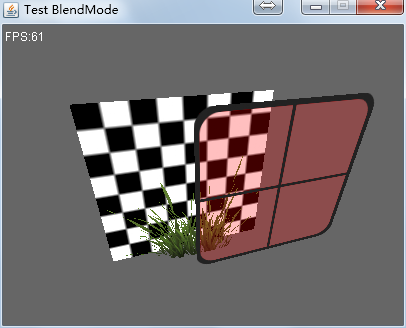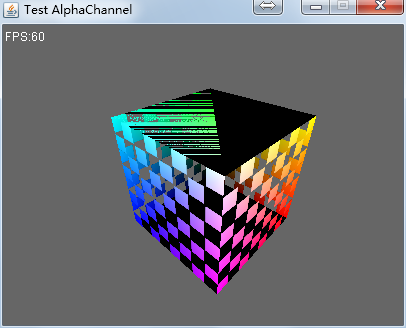### 改进代码

``````(x, y, z, w) + (u, v)
``````

``````(x / w, y / w, z / w, 1 / w) + (u / w, v / w)
``````

#### 改写perspectiveDivide

``````/**
* 透视除法
*/
public void perspectiveDivide() {
float oneOverW = 1f / position.w;
// 透视除法
position.multLocal(oneOverW);
texCoord.multLocal(oneOverW);
// 记录1 / w
position.w = oneOverW;
}
``````

#### 恢复线性插值

(u/w, v/w)，可以直接线性插值了。删除 interpolateLocal 中调用perspectiveCorrect的代码，让所有数据线性插值。

``````/**
* 插值
* @param
* @param v1
* @param t
* @return
*/
public RasterizationVertex interpolateLocal(RasterizationVertex v0,
RasterizationVertex v1, float t) {
// 顶点插值
position.interpolateLocal(v0.position, v1.position, t);

// 法线插值
if (v0.hasNormal) {
normal.interpolateLocal(v0.normal, v1.normal, t);
this.hasNormal = v0.hasNormal;
}
// 颜色插值
if (v0.hasVertexColor) {
color.interpolateLocal(v0.color, v1.color, t);
this.hasVertexColor = v0.hasVertexColor;
}
// 纹理插值
if (v0.hasTexCoord) {
texCoord.interpolateLocal(v0.texCoord, v1.texCoord, t);
this.hasTexCoord = v0.hasTexCoord;
}

return this;
}
``````

#### 修正像素着色阶段

``````public void rasterizePixel(int x, int y, RasterizationVertex frag) {

if (x < 0 || y < 0 || x >= width || y >= height) {
return;
}

// 执行片段着色器
frag.texCoord.multLocal(1f / frag.position.w);// 透视修正

int index = x + y * width;
float depth = frag.position.z;
// ..
}
``````

### 存在的问题

``````/**
* 光栅化三角形
* @param v0
* @param v1
* @param v2
*/
public void rasterizeTriangle(RasterizationVertex v0, RasterizationVertex v1, RasterizationVertex v2) {

// 将顶点变换到投影平面
v0.perspectiveDivide();
v1.perspectiveDivide();
v2.perspectiveDivide();

Matrix4f viewportMatrix = renderer.getViewportMatrix();

// 把顶点位置修正到屏幕空间。
viewportMatrix.mult(v0.position, v0.position);
viewportMatrix.mult(v1.position, v1.position);
viewportMatrix.mult(v2.position, v2.position);

// ...
}
``````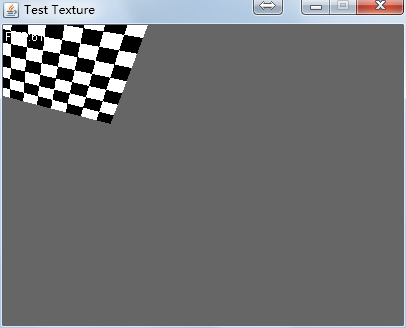#### 原因分析

``````|width/2       0      0    width/2 |
| 0      -height/2    0   height/2 |
| 0            0      1         0  |
| 0            0      0         1  |
``````

``````(x, y, z, w) 变成 (x', y', z', 1)
``````

``````(x' * width/2 + width / 2, - y' * height / 2 + height / 2, z', 1)
``````

``````x = x * width/2 + width/2;
y = -y * height/2 + height/2;
``````

``````f(x, y) = (x, y) * (width/2, -height/2) + (width/2, height/2)
``````

(width/2, height/2)是屏幕的中心点。当x'和y'的取值范围为[-1, 1]时，这个变换的效果就成了先把顶点的原点平移到屏幕中心，然后让x和y的取值在[-width/2, width/2]和[-height/2, height/2]范围内变化。

viewportMatrix * (x', y', z', 1/w)的结果是：

``````(x' * width/2 + width / 2 / w, - y' * height / 2 + height / 2 / w, z', 1 / w)
``````

``````f(x, y) = (x, y) * (width/2, -height/2) + (width/2/w, height/2/w)
``````

x 和 y 的依然在 [-width/2, width/2] 和 [-height/2, height/2] 之间变化，但是现在投影空间的原点不在屏幕中心了！原点被平移到了：

``````(width/2/w, height/2/w)
``````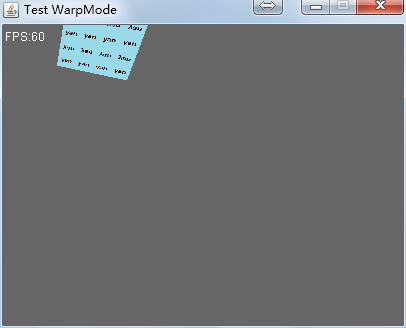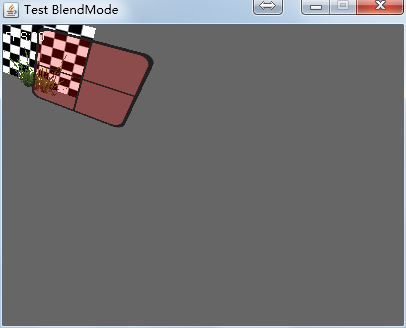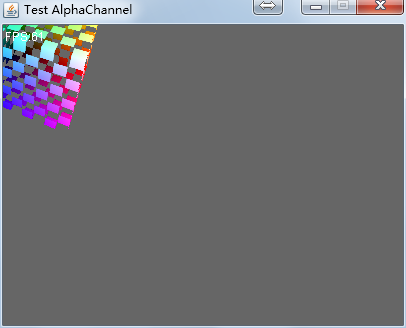#### 解决方法

``````x = x * width/2 + width/2;
y = -y * height/2 + height/2;
``````

``````/**
* 光栅化三角形
* @param v0
* @param v1
* @param v2
*/
public void rasterizeTriangle(RasterizationVertex v0, RasterizationVertex v1, RasterizationVertex v2) {

Matrix4f viewportMatrix = renderer.getViewportMatrix();

// 把顶点位置修正到屏幕空间。
viewportMatrix.mult(v0.position, v0.position);
viewportMatrix.mult(v1.position, v1.position);
viewportMatrix.mult(v2.position, v2.position);

// 将顶点变换到投影平面
v0.perspectiveDivide();
v1.perspectiveDivide();
v2.perspectiveDivide();

// ...
}
``````